# What is Machine Learning?

One of the main differences between humans and computers is that humans learn from past experiences, at least they try, but computers or machines need to be told what to do. Computers are strict logic machines with zero common sense.

That means if we want them to do something, we have to provide them with detailed, step-by-step instructions on exactly what to do.

So we write scripts and programmed computers to follow those instructions. That’s where Machine Learning comes in. Machine learning Concept consists of getting computers to learn from experiences-past data.

# What is Machine Learning?

Machine learning is an application of artificial intelligence (AI) that provides systems the ability to automatically learn and improve from experience without being explicitly programmed. Machine learning focuses on the development of computer programs that can access data and use it to learn for themselves.

The process of learning begins with observations or data, such as examples, direct experience, or instruction, in order to look for patterns in data and make better decisions in the future based on the examples that we provide. The primary aim is to allow the computers to learn automatically without human intervention or assistance and adjust actions accordingly.

# Machine learning methods

Machine learning algorithms are often categorized as supervised or unsupervised.

Supervised Machine Learning algorithms

It can apply what has been learned in the past to new data using labeled examples to predict future events. Starting from the analysis of a known training dataset, the learning algorithm produces an inferred function to make predictions about the output values.

Unsupervised Machine Learning algorithms

In contrast, unsupervised machine learning algorithms are used when the information used to train is neither classified nor labeled. Unsupervised learning studies how systems can infer a function to describe a hidden structure from unlabeled data.

Semi-supervised Machine Learning algorithms

Semi-supervised machine learning algorithms fall somewhere in between supervised and unsupervised learning since they use both labeled and unlabeled data for training — typically a small amount of labeled data and a large amount of unlabeled data. The systems that use this method are able to considerably improve learning accuracy.

Reinforcement machine learning algorithms

Reinforcement machine learning algorithm is a learning method that interacts with its environment by producing actions and discovers errors or rewards. Trial and error search and delayed reward are the most relevant characteristics of reinforcement learning. This method allows machines and software agents to automatically determine the ideal behavior within a specific context in order to maximize its performance.

# Examples of Machine Learning

Machine learning is being used in a wide range of applications today. One of the most well-known examples is Facebook’s News Feed. The News Feed uses machine learning to personalize each member’s feed. If a member frequently stops scrolling to read or like a particular friend’s posts, the News Feed will start to show more of that friend’s activity earlier in the feed.

Behind the scenes, the software is simply using statistical analysis and predictive analytics to identify patterns in the user’s data and use those patterns to populate the News Feed. Should the member no longer stop to read, like or comment on the friend’s posts, that new data will be included in the data set and the News Feed will adjust accordingly.

Machine learning is also entering an array of enterprise applications. Customer relationship management (CRM) systems use learning models to analyze email and prompt sales team members to respond to the most important messages first.

More advanced systems can even recommend potentially effective responses. Business intelligence (BI) and analytics vendors use machine learning in their software to help users automatically identify potentially important data points.

Human resource (HR) systems use learning models to identify characteristics of effective employees and rely on this knowledge to find the best applicants for open positions.

# A blog about explaining k-mean clustering and its real usecase in the security domain

K-means clustering is one of the simplest and popular unsupervised machine learning algorithms.

Typically, unsupervised algorithms make inferences from datasets using only input vectors without referring to known, or labelled, outcomes.

A cluster refers to a collection of data points aggregated together because of certain similarities.

You’ll define a target number k, which refers to the number of centroids you need in the dataset. A centroid is the imaginary or real location representing the center of the cluster.

Every data point is allocated to each of the clusters through reducing the in-cluster sum of squares.

In other words, the K-means algorithm identifies k number of centroids, and then allocates every data point to the nearest cluster, while keeping the centroids as small as possible.

The ‘means’ in the K-means refers to averaging of the data; that is, finding the centroid.

# How the K-means algorithm works

To process the learning data, the K-means algorithm in data mining starts with a first group of randomly selected centroids, which are used as the beginning points for every cluster, and then performs iterative (repetitive) calculations to optimize the positions of the centroids

It halts creating and optimizing clusters when either:

• The centroids have stabilized — there is no change in their values because the clustering has been successful.
• The defined number of iterations has been achieved.

# K-means algorithm example problem

Let’s see the steps on how the K-means machine learning algorithm works using the Python programming language.

We’ll use the Scikit-learn library and some random data to illustrate a K-means clustering simple explanation.

Step 1: Import libraries

`import pandas as pdimport numpy as npimport matplotlib.pyplot as pltfrom sklearn.cluster import KMeans%matplotlib inline`

As you can see from the above code, we’ll import the following libraries in our project:

• Numpy for carrying out efficient computations
• Matplotlib for visualization of data

Step 2: Generate random data

Here is the code for generating some random data in a two-dimensional space:

`X= -2 * np.random.rand(100,2)X1 = 1 + 2 * np.random.rand(50,2)X[50:100, :] = X1plt.scatter(X[ : , 0], X[ :, 1], s = 50, c = ‘b’)plt.show()`

A total of 100 data points has been generated and divided into two groups, of 50 points each.

Here is how the data is displayed on a two-dimensional space:

Step 3: Use Scikit-Learn

We’ll use some of the available functions in the Scikit-learn library to process the randomly generated data.

Here is the code:

`from sklearn.cluster import KMeansKmean = KMeans(n_clusters=2)Kmean.fit(X)`

In this case, we arbitrarily gave k (n_clusters) an arbitrary value of two.

Here is the output of the K-means parameters we get if we run the code:

`KMeans(algorithm=’auto’, copy_x=True, init=’k-means++’, max_iter=300 n_clusters=2, n_init=10, n_jobs=1, precompute_distances=’auto’, random_state=None, tol=0.0001, verbose=0)`

Step 4: Finding the centroid

Here is the code for finding the center of the clusters:

`Kmean.cluster_centers_`

Here is the result of the value of the centroids:

`array([[-0.94665068, -0.97138368], [ 2.01559419, 2.02597093]])`

Let’s display the cluster centroids (using green and red color).

`plt.scatter(X[ : , 0], X[ : , 1], s =50, c=’b’)plt.scatter(-0.94665068, -0.97138368, s=200, c=’g’, marker=’s’)plt.scatter(2.01559419, 2.02597093, s=200, c=’r’, marker=’s’)plt.show()`

Here is the output:

Step 5: Testing the algorithm

Here is the code for getting the labels property of the K-means clustering example dataset; that is, how the data points are categorized into the two clusters.

`Kmean.labels_`

Here is the result of running the above K-means algorithm code:

`array([0, 0, 0, 0, 0, 0, 0, 0, 0, 0, 0, 0, 0, 0, 0, 0, 0, 0, 0, 0, 0, 0, 0, 0, 0, 0, 0, 0, 0, 0, 0, 0, 0, 0, 0, 0, 0, 0, 0, 0, 0, 0, 0, 0, 0, 0, 0, 0, 0, 0, 1, 1, 1, 1, 1, 1, 1, 1, 1, 1, 1, 1, 1, 1, 1, 1, 1, 1, 1, 1, 1, 1, 1, 1, 1, 1, 1, 1, 1, 1, 1, 1, 1, 1, 1, 1, 1, 1, 1, 1, 1, 1, 1, 1, 1, 1, 1, 1, 1, 1])`

As you can see above, 50 data points belong to the 0 cluster while the rest belong to the 1 cluster.

For example, let’s use the code below for predicting the cluster of a data point:

`sample_test=np.array([-3.0,-3.0])second_test=sample_test.reshape(1, -1)Kmean.predict(second_test)`

Here is the result:

`array()`

It shows that the test data point belongs to the 0 (green centroid) cluster.

# Wrapping up

Here is the entire K-means clustering algorithm code in Python:

`import pandas as pdimport numpy as npimport matplotlib.pyplot as pltfrom sklearn.cluster import KMeans%matplotlib inlineX= -2 * np.random.rand(100,2)X1 = 1 + 2 * np.random.rand(50,2)X[50:100, :] = X1plt.scatter(X[ : , 0], X[ :, 1], s = 50, c = ‘b’)plt.show()from sklearn.cluster import KMeansKmean = KMeans(n_clusters=2)Kmean.fit(X)Kmean.cluster_centers_plt.scatter(X[ : , 0], X[ : , 1], s =50, c=’b’)plt.scatter(-0.94665068, -0.97138368, s=200, c=’g’, marker=’s’)plt.scatter(2.01559419, 2.02597093, s=200, c=’r’, marker=’s’)plt.show()Kmean.labels_sample_test=np.array([-3.0,-3.0])second_test=sample_test.reshape(1, -1)Kmean.predict(second_test)`

K-means clustering is an extensively used technique for data cluster analysis.

However, its performance is usually not as competitive as those of the other sophisticated clustering techniques because slight variations in the data could lead to high variance.

Furthermore, clusters are assumed to be spherical and evenly sized, something which may reduce the accuracy of the K-means clustering Python results.

# Real Usecase in the Security Domain:

Clustering is crucial for automating the process of ﬁnding new types of Security Threats that arise during a speciﬁc period of time. The method described later as illustrated in

Figure 3 groups documents that refer to a speciﬁc topic

into clusters and provides a list of the most frequently

occurring terms within each cluster.

Where, J is the objective function, k: is number of clusters, n: is number of cases, c: centroid of cluster j.

The general setting for the cluster algorithm is as follows:

• Clusters the data into k groups where k is predeﬁned.

• Select k points at random as cluster centers.

• Assign objects to their closest cluster center accord-

ing to the Euclidean distance function.

• Calculate the centroid or mean of all objects in each cluster.

• Repeat steps 2, 3, and 4 until the same points are assigned to each cluster in consecutive rounds

Conﬁguration and Speciﬁcation are set, that is most crucial to the operation of the system, is the categorization of the most common security threats, such as DDoS attacks, malware, exploits, and vulnerabilities. This involves the deﬁnition of a set of search terms (keywords) associated with each class o threats. Each deﬁned keyword must have an important level attached to it (weight), denoting the contribution of an occurrence of this word to the score of each document (I). These keyword lists must follow the format as follows:

I. Keywords related to possible attacks.

1. Denial of Service (DoS) : ddos attack, take down website,server / computer crush, server take down

3. Account hijacking : account hacked ,account images changed hack,take control account,account add/remove content

Threat Class 1:

keywords: {[keyword1, weight], [keyword2, weight]…

[keywordN, weight]}

• Threat Class 2:

keywords {[keyword1, weight], [keyword2, weight]…

[keywordN, weight]}..

• Threat Class N:

keywords: {[keyword1, weight], [keyword2, weight]…

[keywordN, weight]}

The simulation of the proposed system is conducted based on the crime pattern analysis. The crime pattern analysis can be deﬁned as an analytical technique that gives relevant information in regard to the crime patterns . The simulation process is accomplished by conducting k-means clustering. The k-mean clustering is performed on crime data sets with the use of rapid data tool. The simulation is carried out in steps. Firstly, a data set is obtained.

Secondly, the obtained data set is ﬁltered according to the requirements, and then, a new data set with the attributes according to the analysis to be conducted is created.

Thirdly, an open minor tool is opened and then the excel ﬁle read. The “Replace the Missing value” operator is then applied, and then the operation executed. Fourthly, the “Normalize operator” is performed on the resulting data set and then operation executed. Finally, k-means clustering is performed on the resultant data set after the normalization process.

Finally, k-means clustering is then performed on the resultant data set after the normalization process. The analysis is then done on the cluster formed. The created approach was tested with few thousands of fake documents containing random computer terminology, using a free python script called “B. Generator”. The script was slightly modiﬁed to include some extra keywords to produce around 10000 documents to be stored in speciﬁc database. For clustering a number of collected documents within the database in k categories, we could use the k-means Algorithm, also known as Lloyd’s algorithm.

# Conclusion:

he future work should focus mainly on the implementation of a full system and should aim to integrate the proposed system with the appropriate portal, currently being worked on by several IT companies. Once the proposed system is fully developed, it can be used to collect some amount of data over a speciﬁed time so that it can be used to establish the data mining algorithms. The proposed system can also be used for training purposes. For instance, when the system is properly implemented, it can be used as a benchmark by any related future works.

By exploring the various IT technologies, other pro- gramming languages in Latin scripts, such as Korean, Russian, Greek, and Chinese, can be added. To this end, the best format to be used to present the security alerts has not been determined. Therefore, the future works should determine the best alert presentation format to enable the security agents to respond to the detected threats within the shortest time possible. After the proposed system has been set up and tested, it should be developed by adding new features to improve the communication among the modules. The new features that may be added include triggering mechanism or message brokers that will notify the system of any breakdown in any module.

The vast amount of data generated in the Internet era undoubtedly challenges the technology of large-scale data processing and data mining. In this paper, we study the problem of network security by using k-means clustering algorithm in data mining. Analyses the network security problems and performance better intrusion detection system in network security analysis simulation, let more people know the network intrusion behavior produces a variety of ways and means. In this way, we can ensure the security of the network information in the network information leak serious today.

--

--

--

Flutter Developer & Full Stack Developer

Love podcasts or audiobooks? Learn on the go with our new app.

## What is Deep Learning: Basics That Every Beginner Should Know## Using NLP and LSTM to combat cyberbullying## Recognizing Handwritten Digits with Scikit-Learn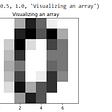## Maximizing Business Impact with Churn Prediction Using Machine Learning: A Practical Approach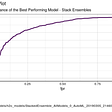## Building Mario Levels with Machine Learning## Running ML Python Code in Parallel With RAY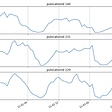## Machine Learning made Easy — Linear Regression An Intuition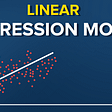## Performance Metrics: Receiver Operating Characteristic (ROC)- Area Under Curve (AUC)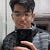Flutter Developer & Full Stack Developer

## Sable: Glide of Passage.## Tutorial: training a dependency parser model in Stanford Stanza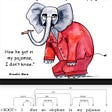## Enough Already: End Greenwashing Now